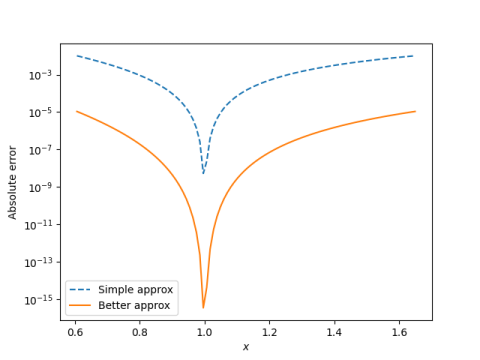# Better approximation for ln, still doable by hand

A while back I presented a very simple algorithm for computing natural logs:

log(x) ≈ (2x – 2)/(x + 1)

for x between exp(-0.5) and exp(0.5). It’s accurate enough for quick mental estimates.

I recently found an approximation by Ronald Doerfler that is a little more complicated but much more accurate:

log(x) ≈ 6(x – 1)/(x + 1 + 4√x)

for x in the same range. This comes from Doerfler’s book Dead Reckoning.

It requires calculating a square root, and in exchange for this complication gives about three orders of magnitude better approximation. You could use it, for example, on a calculator that has a square root key but not log key. Or if you’re dead reckoning, you need to take a square root by hand.

Here’s a plot of the error for both approximations.The simpler approximation has error about 10-2 on each end, whereas Doerfler’s algorithm has error about 10-5 on each end.

If you can reduce your range to [-1/√2, √2] by pulling out powers of 2 first and remembering the value of log(2), then Doerfler’s algorithm is about six times more accurate.

By the way, you might wonder where Doerfler’s approximation comes from. It’s not recognizable as, say, a series expansion. It comes from doing Richardson extrapolation, but algebraically simplified rather than expressing it as an algorithm.